AIRFOILS 3D ROTATIONS IN LEPARAGLIDING
Study of geometric transformations to rotate airfoils in three axes

3. A bit of analytical geometry in space
4. Graphic OpenSCAD experimentation and study of the movements and their compositions
5. Angle phi between line and plane
6. Angle chi between two lines in space
7. Example

1. Motivation

Define geometrically a paraglider wing, basically is to locate airfoils in the space with the appropiate rotations, traslacions, and factors of the scale. Program LEparagliding until versions 3.15 rotates the airfoils in two axes:
- First rotation on an axis perpendicular to the profile plane (axis parallel to "X"), to achieve the desired relative angle of incidence "washin" assuming the wing is flat and the airfoils parallel to each other.
- Second rotation on an axis coinciding with the profile chord before the first rotation, (axis parallel to "Y")
This system is simple, works, and allows us to define excellent wings. However, some time ago I think in a third rotation, a rotation around the vertical axis "Z", which implies that the ribs are not all parallel to each other in the planform scheme.

Talking with Arnaud Martinez about his new design, the Stork, made me realize that the additional rotation around "Z" axis can be useful to insert the airfoils within the glide trajectory. That is to say, to ensure that the line of trajectory is contained in the airfoil plane, so the flow will cross the airfoil according to the calculated shape. Of course, we can adjust the angles in the "Z" to obtain other effects.

So soon we will add two additional columns to define the geometry of the wing in SECTION 1, one for the angle Z, and the other to set the position of the axis of rotation with respect to the % chord...Figure 1. This scheme is used to realize the importance of aligning the airfoils according to the glide path. The central airfoil (A) is in a vertical plane, and therefore the trajectory of the flight is contained within. The wingtip airfoil (C), may be in a completely horizontal plane. Thus, it is not well inserted into the glide path. If we do a rotation equal to the glide angle in an horizontal axis (vertical local axis of the airfoil), we get the glide path inside the airfoil plane. So the aerodynamic performance will be maximum. For a generic airfoil (B) it will be necessary to do an intermediate rotation.

2. Airfoil rotations in space

To add a third rotation in the space, I have to check the code currently used by the program, and especially to analyze in what order to apply the rotations and with what reference points do not create a mess. This is what I'm currently doing.

We consider the orthonormal axes x,y,z as the local airfoil axes content in the plane yz. The rotated airfoil will have the local axes x',y',z'. And the three rotations to consider are the following:Figure 2

3. A bit of analytical geometry in spaceFigure 3. Definition of the director cosines, used in the matrix of rotation of coordinate systems.Figure 4. General transformation of coordinates in the space, by rotation and translation.Figure 5. We obtain the coordinates transformation in the case of a rotation around an axis parallel to Z.Figure 6. We obtain the coordinates transformation in the case of a rotation around an axis parallel to X.Figure 7. We obtain the coordinates transformation in the case of a rotation around Y axis.

Well, now we have the analytic expressions for the coordinate transformation for any of the rotations. The next step is to define the order of application of the rotations and with respect to which axes. This is important to ensure compatibility with previous versions of LEparagliding and not to get strange results. When Z-rotations are defined as zero, the results of the 3D model must be exactly the same as in previous versions.

4. Graphic experimentation and study of the movements and their compositions, using openscad

Before applying the rotation formulas, it is necessary to analyze in detail what is the effect of each of the rotations with respect to the axes considered and how influences the order of application. We modeled a gnuLAB3.dxf profile with the OpenSCAD program and visualized the movements. OpenSCAD is ideal for doing graphical experiments of this type. Here the OpenSCAD script and all files in .zip fomat here.01 Rotation X 20 deg 0%. Gray = airfoil initial. Red = rotation about X axis.02 Rotation X 20 deg 50%. Red = rotation about an axis parallel to X and located at 50% of chord.03 Rotation X 20 deg 100%04 Rotation Y 90 deg local 3D (yellow airfoil)05 Rotation Y 90 deg local view YZ06 Rot Y 45 deg. Usually the rotations aroud Y will be between 0 and 90 degrees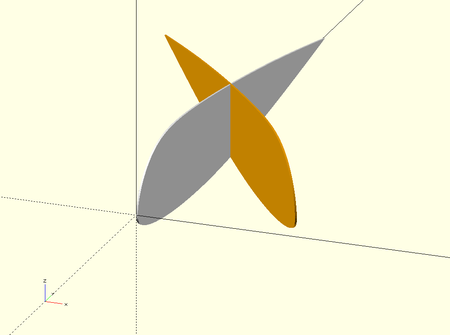07 Rotation Z 35 deg local 3D. Orange = Rotation around a vertical axis. The ribs are not parallel.08 Rotation Z 35 deg local top view XY.09 Rot Z 35 deg 20%. The position of the vertical axis of rotation can be adjusted along the chord.10 Rotation X 20 deg and Y 0 and Z local 3D. Rotation of the profile around its local vertical Z-axis (local axis perpendicular to the chord of the red profile, which already has an angle of washin).11 Rotation X 20 deg and Y 0 and Z local postion 100%12 Rotation X 20 deg and Y 0 and Z local view yz. Here we can see clearly that the rotation in Z, it is with respect to an local axis, not the global Z axis. If we rotate around the global Z axis the results are others. For this reason, it is important to pay attention on which are the axes of rotation (global or local to the previous trasformed airfoil).13 Rotation X 20 deg and Y global 90. Green = 90 degrees rotation with respect to the global Y-axis. This is the axis of rotation in Y, which we use in LEparagliding.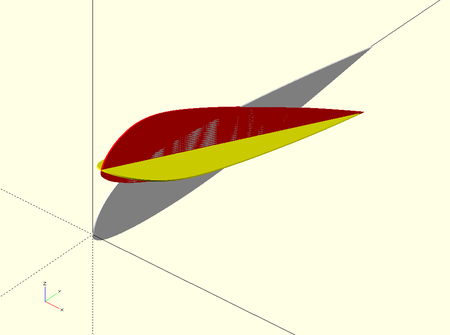14 Rotation X 20 deg and Y local 90. Yellow = Red airfoil turned 90 degrees with respect to its local Y-axis. The results are very different from those of the previous case.15 Rotation X 20 deg and Y local (yellow) and global (green) 90 degrees.16 Rotation X 20 deg and Y local-global 90 top view XY.17 Rotation X 20 deg and Y local-global 90 and Z local. If we add a rotation to the green airfoil arount its vertical local Z-axis, we obtain the orange airfoil, which is what we want to target with the flow...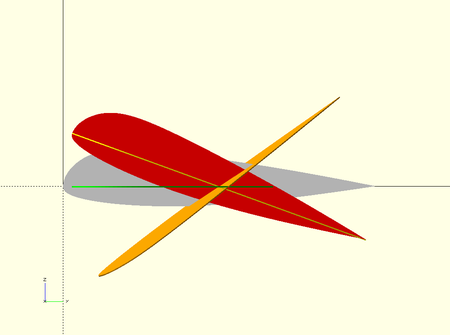18 Rotation X 20 deg and Y local-global 90 and Z local, view YZ. The orange profile, represents a wingtip profile, which preserves the desired angle of attack and by a rotation around its vertical axis.19 Rotation X 20 deg and Z global 35 view YZ. Compare with figure 1220 Rotation X 20 deg and Y local-global 90 and Z. The orange profile, represents a wingtip profile, rotated in local axis. The blue profile, represents a wingtip profile, rotated in global axis.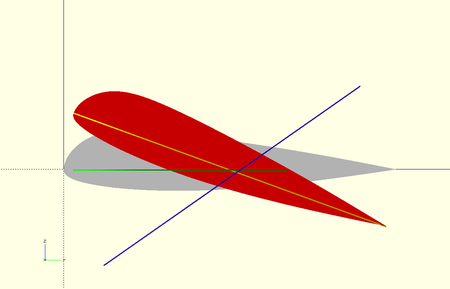21 Rotation X 20 deg, Y local-global 90, and Z global, view YZ. The blue profile, represents a wingtip profile, which preserves the desired angle of attack and by a rotation around global vertical axis is placed in the glidepath... maximizing the aerodynamic performance. Note: the angles in these figures are exaggerated for better visualization.
Figure 8. Graphical rotations experiments with OpenSCAD

The conclusion of this graphical experiment is that the best strategy is the one shown in subfigure 8-21:

- The first rotation is applied on an axis parallel to X global, intersects the chord of the initial profile (grey) at a distance between 0 and 100% of the nose
- Then a second rotation is applied on an axis parallel to Z global, intersects the chord of the initial profile (grey) at a distance between 0 and 100% of the nose
- And the third rotation is applied around the Y-axis global

Three rotations on these axes and exactly in this order to get the desired results. Easy!

5. Angle phi between line and plane

To know how the additional rotation in Z influences, now we want to know what is the angle phi formed between the profile plane and the glidepath line... The answer is found very easy, in the Handbook of Mathematics by I. Bronshtein and K. Semendiaev "Справочнике по математике для инженеров и учащихся втузов. И. Бронштейн, К. Семендяев".Figure 9.
Angle phi between line and plane.

In the calculation introduced in the program LEparagliding, we have used an inclination of the glidepath equal to the angle of attack alpha calculated in the central profile, because we are comparing the glidepath trajectory with the plane of the profiles referred to the XYZ coordinate system, which in equilibred flight, rotates around the Z axis at a tetha angle equal to the assiette parameter. If the flight angle with respect to the horizontal line is gamma, it is verified that alpha + tetha = gamma . View angles here.

6. Angle chi between two lines in space

Now we want to know the "perceived angle of attack" (local angle of attack) for each of the profiles considering their orientation in space. To do this, we will consider in a simplified way, the angle chi formed between the profile chord line and the glide path line. The result will be closer to the exact local angle of attack, when the plane of the profile is more aligned with the trajectory, since the two lines do intersect at a point.

Once again, we find a very elegant formula to calculate this, in the book of
I. Bronshtein and K. Semendiaev "Справочнике по математике".Figure 10. Angle between two lines in space, based on their director cosines.

OK! Now we have all the formulas to update the program code! :) Rotations in space have been introduced in sections 6.5, 12.3, 12.4, 14.3, and V-ribs 16.1,16.2,16.3,16.4,16.6. The calculation of the angle between the glidepath and profiles, and local angle of attack in section 10.1+. The amount of code required is small, but the result is spectacular!

7. Example

We have created a leparagliding.txt data file for version 3.16 of the program, a theoretical wing for instructional purposes only. It is not a real wing. The torsion angle parameters are exaggerated and the number of cells is too small. The only goal is to understand how the profiles rotate in space, and to verify that the program works as expected. Download all example files here including DXF. Commented screeshots:

Model M1 = model with Z rotations equal to 0 in column 10 of leparagliding.txt SECTION 1
Model M2 = model with Z some rotations not 0 in column 10 of leparagliding.txt SECTION 1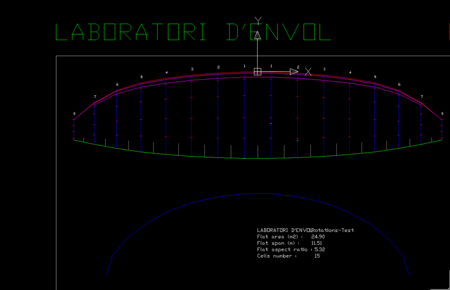01 M1 Planform and vault. All rins are parallel.02 M1 Planform 3D. Attention! In this model, all the ribs still are parallel to each other. The apparent lack of parallelism is due to the effect of the geometric torsion (washin).03 M1 Lateral view. Notice the wingtip profile, located in a completely horizontal plane. So poorly oriented to the trajectory.04 M1 Wingtip washin 30 deg.05 M1 Wingtip and glidepath06 M1 Trajectories07 M1 planform and trajectories08 M2 front view09 16 M1-M2 wingtip zoom10 M2 2D. Notice the new yellow lines, with the rotations on the vertical axis Z incorporated11 Model M1 using rotations i Z=0.11 Model M2 using rotations in Z not 012 M2 Notice wingtips perfectly oriented within flow. The other profiles are also on the trajectory line! :)13 M2 and M1. 3D overlay of models. The M2 profiles rotated in Z (yellow color), have more angle than the others M1 (green-blue) .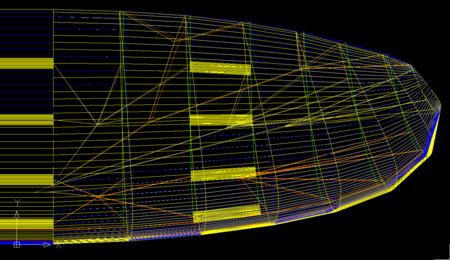14 M2 (yellow) and M1 comparative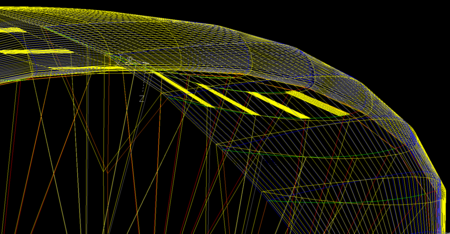15 M1-M2 3D comp. Apparently, the angles of attack in M1-M2 are similar. Topic to be studied in more detail.
Figure 11. Some screenshots, comparing a model with parallel profiles (M1) and a model with profiles better aligned to the flow (M2).

When we run the program using profiles with rotation values "0" around the Z axis (model traditionally used by LEparagliding), we obtain the angles phi between the glidepath line and the plane of the profiles, which now are listed with informative purposes in section 12 of the lep-out.txt file:

12. ANGLES BETWEEN AIRFOIL PLANE AND GLIDEPATH LINE  (phi) and local AoA (chi)
--------------------------------------------------- -------------------------
Informative angles. Change rotation values
in column 10 of SECTION 1, using phi to improve the results
phi=angle between airfoil plane and glidepath line
chi=angle between chord line and glidepath line (local AoA)

Rib    phi      chi
1    -0.00    11.31
2     1.95    14.27
3     2.91    17.17
4     4.75    20.80
5     5.63    25.42
6     7.97    29.07
7     8.64    33.34
8    11.31    31.87

The angles phi in profiles 1 and 2 are intuitive and are 0 or almost zero since the profiles are in a vertical plane. The angle in profile 8 is also intuitive, it is exactly the angle of attack alpha since we have defined profile 8 in an XY plane. If we want the profile 8 to be inserted in the glidepath line, we have to rotate exactly phi on the Z axis. For the other values it is less intuitive, but if we apply a rotation Z in column 10 of the geometry matrix exactly equal to phi , for each one of the profiles and run again the program we obtain lep-out.txt:

12. ANGLES BETWEEN AIRFOIL PLANE AND GLIDEPATH LINE  (phi) and local AoA (chi)
--------------------------------------------------- -------------------------
Informative angles. Change rotation values
in column 10 of SECTION 1, using phi to improve the results
phi=angle between airfoil plane and glidepath line
chi=angle between chord line and glidepath line (local AoA)

Rib    phi      chi
1    -0.00    11.31
2     0.04    14.14
3     0.05    16.92
4     0.08    20.24
5     0.08    24.78
6     0.08    27.97
7     0.07    32.24
8    -0.00    30.00

OK! This means that the plane of the profiles exactly matches the glidepath line! (values of + -0.1 deg are not significant). That's what we wanted to achieve! :)

Now, we now very easily how to force the program to automatically rotate the profiles around Z-axis to align with the glide path. But it is better to write the recommended results in the lep-out.txt file and let the designer decide what the best values are. Since some parameters like the alpha angle are very debatable. And Z-rotations can be done with other criteria according to each designer.

In this section, we have added the result of the calculation of the local angle of attack chi "seen" by profiles, also with informative purpose.

The conclusion is that rotating the profiles in Z is not just an aesthetic issue. If done with the correct angles, the performance of the wing can be increased as the profiles will work with their best aerodynamic shape.

Pere Casellas

Teià, August 22 2021